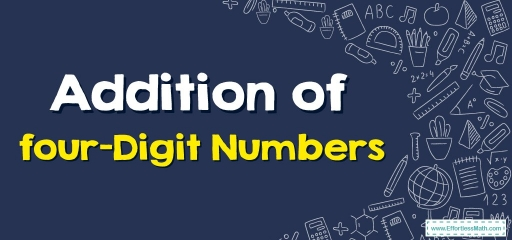The four-digit numeral series starts with $$1,000$$ then the last one is $$9,999$$. Four-digit numerals are numerals with four digits and it’s possible to create a four-digit number via the use of any kind of number zero to $$9$$, although the numeral has to start with the digit $$1$$ else a numeral higher than one.

If two $$3$$-digit numbers are added, after that the precise method is used for adding up four-digit numbers!

## How to do addition of four-digit numbers?

Step one: The column technique requires putting one figure under the other one. Therefore, in this instance, the hundreds’ spot digits, the tens’ spot digits as well as ones’ spot digits all lineup. Put these numerals into a column, and always begin the addition of the ones first. Place a plus sign $$(+)$$ to the left of these numbers. As an alternative to the equal sign $$(=)$$, place a line under the lower amount.

Step two: Add these numbers, beginning from the right side. Add up the two ones’ spot digits then put the result under the line.

Note one: If $$2$$ numbers within a place value column get added up and equal a number higher than $$9$$, then you have to carry a number. Don’t forget to add on the numeral that was carried over.

Step three: Add all numerals in the tens’ spot column, then put the result under the line as well as to the left of the ones’ spot amount.

Step four: Add up the numerals in the hundreds’ spot column, then put the result under the line as well as to the left of the tens’ spot amount.

Step five: Do the same for numerals in the thousands’ spot column and put the result under the line as well as to the left of the hundreds’ spot amount.

Note two: When doing the addition of four-digit figures, you must first estimate then check after – it is a great idea to approximate a rough answer, then check the real result.

Note three: When doing addition of four-digits figures, order does not matter – for instance, don’t forget $$532 + 245$$ equals $$245 + 532$$.

### Addition of Four-Digit Numbers – Example 1:

Find the sum.

$$\begin{array}{r} &3,520\\ +\!\!\!\!\!&1,435\\ \hline \end{array}$$

Solution:

First line up the numbers: $$\begin{array}{r} &3,520\\ +\!\!\!\!\!&1,435\\ \hline \end{array}→$$ Add the numerals in the ones’ spot column $$(0 + 5 = 5)$$ and put the resulting answer under the line. $$\begin{array}{r} &3,520\\ +\!\!\!\!\!\!&1,435 \\ \hline \end{array} \\ \ \ \ \ \ \ \ \ \ \ \ \ \ 5$$ , Continue with tens’ place. $$2 \ + \ 3=5$$, $$\begin{array}{r} &3,520\\ +\!\!\!\!\!\!&1,435 \\ \hline \end{array} \\ \ \ \ \ \ \ \ \ \ \ \ 5$$. Add the numerals in the hundreds’ spot column then out this answer under the line, $$5 \ + \ 4=9$$, $$\begin{array}{r} &3,520\\ +\!\!\!\!\!\!&1,435 \\ \hline \end{array} \\ \ \ \ \ \ \ \ \ \ \ 9$$. Add the numerals in the thousands’ spot column then put this answer under the line, $$3 \ + \ 1=4$$ $$\begin{array}{r} &3,520\\ +\!\!\!\!\!\!&1,435 \\ \hline \end{array} \\ \ \ \ \ \ \ 4,955$$

### Addition of Four-Digit Numbers – Example 2:

Find the sum.

$$\begin{array}{r} &4,384\\ +\!\!\!\!\!&2,613\\ \hline \end{array}$$

Solution:

First line up the numbers: $$\begin{array}{r} &4,384\\ +\!\!\!\!\!&2,613\\ \hline \end{array}→$$ Add the numerals in the ones’ spot column $$(4 + 3 = 7)$$ and put the resulting answer under the line. $$\begin{array}{r} &4,384\\ +\!\!\!\!\!\!&2,613 \\ \hline \end{array} \\ \ \ \ \ \ \ \ \ \ \ \ \ \ 7$$ , Continue with tens’ place. $$8 \ + \ 1=9$$, $$\begin{array}{r} &4,384\\ +\!\!\!\!\!\!&2,613 \\ \hline \end{array} \\ \ \ \ \ \ \ \ \ \ \ \ 9$$. Add the numerals in the hundreds’ spot column then put this answer under the line, $$3 \ + \ 6=9$$, $$\begin{array}{r} &4,384\\ +\!\!\!\!\!\!&2,613 \\ \hline \end{array} \\ \ \ \ \ \ \ \ \ \ \ 9$$. Add the numerals in the thousands’ spot column then out this answer under the line, $$4 \ + \ 2=6$$ $$\begin{array}{r} &4,384\\ +\!\!\!\!\!\!&2,613 \\ \hline \end{array} \\ \ \ \ \ \ \ 6,997$$

## Exercises for Addition of Four-Digit Numbers

Find each sum.

1. $$\color{blue}{5,338+2,541}$$
2. $$\color{blue}{7,542+1,329}$$
3. $$\color{blue}{3,875+3,161}$$
4. $$\color{blue}{6,462+2,525}$$
1. $$\color{blue}{7,879}$$
2. $$\color{blue}{8,871}$$
3. $$\color{blue}{7,036}$$
4. $$\color{blue}{8,987}$$

### What people say about "Addition of Four-Digit Numbers - Effortless Math: We Help Students Learn to LOVE Mathematics"?

No one replied yet.

X
30% OFF

Limited time only!

Save Over 30%

SAVE $5 It was$16.99 now it is \$11.99